# Understanding Gyroscopes

## A deep dive into the intuition and mathematics of why Gyroscopes seemingly defy gravity.

yroscopes are used in spaceships to navigate, and they are probably in your smart phone as well allowing you to do cool stuff, which is why you want to understand how they work.

Now there are lots of different videos and writings out there trying to explain gyroscopes to you, so why read this one? I find that people tend to either try to simply show you the math and then basically assume that makes it all obvious to you or they try to convey some kind of intuition about why it works the way it does.

Instead I will try to do both, and I will not make too many assumptions about what you already know about math. There is a tendency to overstimate just how much math people remember or know.

# Understanding Torque

If you want to understand gyroscopes, then you have to understand torque. Torque could be thought of as a rotational force: a force that causes or inhibits rotation.

## The Math of Rotating Stuff

Torque relates to stuff rotating, so it helps to understand torque by first looking at equations describing rotational movements. Alternatively you can think of this as equations for things moving in orbits or circles.

I’ll do this by comparing the equations for rotational movement with the better known one for linear motion. Movement along a straight line in other words.

In all my examples I will assume acceleration is either zero or constant.

## Linear Motion

If a constant acceleration a is applied to an object initially at rest for the time t then it will get the velocity v. This should be familiar to you if you did physics in high school.

`v = at`

## Newton’s second law

The acceleration an object of mass m gets when a force F is applied to it, is proportional to the force, and inverse proportional to its mass.

`a = F/m`

Let me give you an example. If you throw a snowball, you will apply a force F with your hand on the snowball with mass m. That will give the snowball an acceleration of a.

This equation is called Newton’s second law, and is usually written on this form.

`F = ma`

Which could be stated as the net force F applied to an object is proportional to the acceleration and mass of the object.

Or with our snowball example. The force you’re hand pushed on the snowball is equivalent to mass and acceleration of the snowball. If the snowball gets a quick acceleration, then either the force applied by your hand needs to be higher or the mass of the snowball smaller.

## Newton’s third law

One of Newton’s observations which doesn’t seem quite obvious at first is that

for every force, there is a force working in the opposite direction.

So if you stand on super slippery sheet of ice and push your friend, you will both move backwards.

Your friend doesn’t need to actively do anything to apply this force to you. You could replace your friend with a curling stone and push on that. The result would still be that you and the stone would be pushed in opposite direction.

This is an important principle to understand in rocketry, since this is how a rocket moves. A way to simulate a rocket is to load yourself up with lots of snowballs, stand on the ice and start throwing them. If your shoes and ice is slippery enough you will gain some acceleration in opposite direction of where you are throwing snowballs each time. In a sense your snowballs are working as your rocket propellant.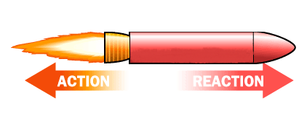Newtons 3rd law says: for every action, there is an equal and opposite reaction. A force from the propellant pushes on the rocket and the rocket push back with an equal but opposite force on the propellant.

If you got a mass m₁ and obtain an acceleration of a₁ when throwing a snowball of mass m₂ getting an acceleration of a₂, then there will be a force F causing this accelerations. We can express this with the two equations.

`F = m₁a₁F = m₂a₂`

Which means we could write

`m₁a₁ = m₂a₂`

Further lets say you spend Δt time throwing the snowball giving it a velocity of v₂ by the time it exits your hand, while your are accelerated to a velocity of v₁ in the opposite direction. We could then make the substitutions a₁ = v₁/Δt and a₂ = v₂/Δt, to get:

`m₁v₁/Δt = m₂v₂/Δt`

This can be simplified by eliminating Δt on both sides:

`m₁v₁ = m₂v₂`

Hence we have arrived at an important principle in mechanics. The quantity mv is important since it is preserved, when objects interact, whether you are throwing snowballs or billiard balls hit each other. So it has gotten its own name which is momentum.

`p = mv`

## Rotation

Let’s take what we have learned about linear motion and compare with circular movements. Instead of talking about distances in meters, we deal with distances in angles. Like how many degrees or radians and object has moved.

The arch length, is the distance between two points on a circle following the curvature of the circle. So no shortcuts by using a straight line. For the full circle the arch length is the same as the circumference:

`S = 2πr`

When measuring angles, using radians, we are essentially stating angles in terms of number of radii. So the total number of radians in a circle is:

`θ = S/r = 2πr/r = 2π`

Angular velocity ω could be expressed as a change in angle per time unit δt

`ω = δθ/δtω = δ(S/r)/δtω = δS/rδt`

And since δS/δt just describes a tiny displacement per tiny time interval, that is just the same as velocity, so we get:

`ω = v/r`

We can derive equations for angular acceleration α, first with relation to angular velocity.

`v = atv/r = at/rω = αt`

But we could also derive its definition by:

`α = δω/δtα = δ(v/r)/δtα = δv/rδtα = a/r`

Just like we got momentum p = mv for linear motion, we got angular momentum:

`L = Iω`

where I, is the rotational inertia, serving the same purpose in rotation based equations as mass for linear motion. Both give inertia or resistance to a force trying to change an objects’ motion.

We also have a similar relation as F = ma for rotation, which is that torque τ is proportional to rotational inertia and angular acceleration

`τ = Iα`

The relationship between torque and force is

`τ = rF`

I am skipping some details here as I am mainly trying to build an understanding rather than allowing you to perform all the calculations on examples yourself. One of those it that torque is a vector. But I kind of assume you already have been exposed to this concept.

## Why Torque is Vector

A common misunderstanding is to think torque is its own special thing. But it is really just a higher level explanation of the interactions of regular forces on objects in a manner which causes them to spin around.

Think of heat. Heat is just a higher level description of the kinetic energy of all the molecules of the substance being hot.

If I hold a bolt with a wrench and pull on it in the counter clockwise direction, then I will apply a torque going down through the rotating axis of the bolt. This is according to the right hand rule, which says if I hold my right hand in the direction of the radius and then bend my fingers in the direction of the force I am applying (with the wrench in this case), then my thumb will point in the direction of the torque.

If you are like me when first learning this, you might find this very odd. It sounds like torque is a special force, having a completely different direction from the force I am applying to the wrench.

But that isn’t what is going on at all. Torque is just a pseudo-vector. There is no actual special kind of force going in the direction of the torque vector. The torque vector is just a convenient way of representing torque. We could have made it into a curved arrow instead, where the length represented the magnitude of the force, and the direction of the arrow indicate which direction the torque was applied.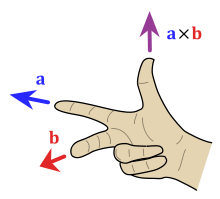When doing the cross product between two vectors, the right hand rule gives us the direction of the result. To get correct direction (according to established conventions) for torque we do τ = r×F

Nor is there anything special about the right hand rule. That is just a convention as well. The Soviets e.g. until the 1960s used a left hand rule instead. That also works. Now you might argue, that if we used a left hand rule, then the bolt would move the opposite direction of the torque, when pulling on the wrench.

True, but the torque that will make a bolt go down or up is also just a convention. Bolts are made that way. They could have been made to go down when pulling the wrench in the counter clockwise direction as well. In fact at the crank gear of your bicycle, on one of the sides, the bolt will act just this way.

So it doesn’t really matter which direction you draw a torque vector. The point is that whatever you do, you have to be consistent. If you use the right hand rule to determine the direction of one torque then you have to do it as well for all other torques and when you are dealing with angular velocity, angular acceleration and angular momentum.

This is so that if I see somebody draw a vector representing angular acceleration, then by using the right hand rule I should be able to find the direction of that acceleration, whether it is clockwise or counter clockwise. The direction of that acceleration should be the same as the force that was applied to get that acceleration. The torque vector of that force has to point in the same direction as the angular acceleration vector of the acceleration. If not we would quickly confuse ourselves.

Another way to think of the vectors for torque, angular acceleration or angular velocity, is to think of how 2D planes are often represented in mathematics. A 2D plane is usually represented as a 3D vector perpendicular to the plane.

Now it might seem odd with the vector pointing in a direction where the plane isn’t, but we are really just using the vector to describe the orientation of the face of the surface. A vector like that does not “look” like the plane it represents. But that is irrelevant in mathematics. What we care about is whether we can use the vector to make useful calculations related to a plane. And we can.

We can use it to check if two planes are intersecting or not, for instance, by checking whether their corresponding vectors are parallel or not. Finding the angle between the vectors would find the angle the planes would make at their intersection.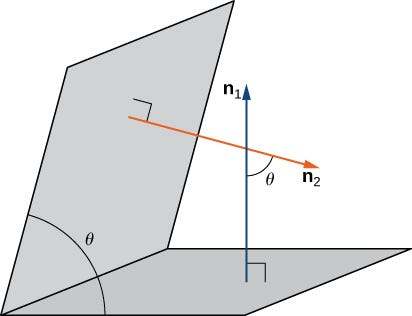We can use the normal vectors n₁ and n₂ of two planes to find the angle θ of their intersection.

Likewise a torque vector doesn’t “look” like the torque, but it allows anybody to derive important information about the actual torque. You can look at the length to find the magnitude of the torque and you can use the right hand rule in reverse to find out where the force was applied. It also tells us axis it will spin around, if the object was initially at rest.

## Why is Torque Affected by the Radius

We sort of understand this from practice. It is easier to turn a bolt with a long wrench than a short one. So somehow we can trade in force for longer radius. But how do we explain this in a mathematical fashion?

Let us say we push on a ball with mass m attached to a rigid metal arm with a force F. This will give it an acceleration a.

`F = ma`

We can can express a as αr

`F = mαr`

Which gives us an expression for angular acceleration for a given mass, radius and force.

`α = F/(mr)`

Let us say we push on the arm at different radiuses r₁ and r₂, but we push such that they get the same acceleration α

`α = F₁/(mr₁)α = F₂/(mr₂)F₁/(mr₁) = F₂/(mr₂)`

Removing common factors on each side we are left with

`F₁/r₁ = F₂/r₂`

This gives us a hint that it is a combination of radius and force which affects the acceleration a rotating object gets.

But to derive that τ = rF, we’ll look at another example. Work W is defined as the constant force you F you use to move an object a distance s:

`W = Fs`

I explain how this relationship between work and forcer is derived here.

Recall earlier example: We push at different points on the right metal arm, r₁ and r₂. It is possible to substitute s for the arch length of a circle. Remember the relationship:

`θ = S/r`

This can be rewritten to say that the arch length S is the product of the angle in radians and the radius:

`S = θr`

We know when pushing on the metal arm, that we want to perform the same amount of work, and move the same number of degrees. However the radius will be different and we assume we don’t know if they force will be different.

`W = θr₁F₁W = θr₂F₂θr₁F₁ = θr₂F₂r₁F₁ = r₂F₂`

So we conclude that when pushing an object in circles, it is the product of force and radius which affects its motion. As with momentum, because this product is so important we give it a name, which is torque, denoted with a τ.

# Why Do Spinning Tops Not Tip Over?

Remember a gyroscope is just a spinning top embedded in some gimbals, so to understand gyroscopes lets discuss a spinning top first.

If a top isn’t spinning it tips over. With a fast spin, it will take less time to tip over than when it is given less angular velocity. We can observe this phenomenon with many different objects. Your bike doesn’t tip over if you wheels have a high angular velocity. That is why you can make sharp turns leaning over as long as you go fast.

You can spin a ball attached to rope over your head and the faster you spin it around, the more it seems to defy gravity.

The centrifugal governor of a steam engine, also utilize this principle.

It is also somewhat related, the reason why a satellite doesn’t crash down on the earth despite constantly being pulled on by the earth towards its center. As with the top, if the satellite didn’t go fast enough around the earth it would have fallen down to the ground on earth.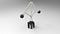A centrifugal governor usually used to regulate the flow of fuel to engines. The black balls will begin to rise up as the contraption spins faster.

Lets consider a centrifugal governor. The balls attached by metal arms to its central spinning axis, should be moving in straight lines under normal circumstances. When they instead are moving in circles, that means there is a centripetal force, accelerating the balls towards the center. This acceleration is defined by the equation:

`a = v²/r`

Listen to Sal at Khan Academy for a great explanation of how we arrive at this equation. I’ll do a quick recap of his video.

`s = vtt = s/v`

For circular movements the time it takes to travel around it is thus the circumference divided by velocity:

`t = 2πr/v`

Sal showed how we can imagine a similar circle where we replace radius with a velocity vector, and the velocity vector with an acceleration vector.

`t = 2πv/a2πr/v = 2πv/ar/v = v/a`

Which gives us

`a = v²/r`

The acceleration vector a will be pointing towards the rotation axis along the metal arms holding the balls, which means it is pointing slightly upwards. It isn’t merely lying in the horizontal plane. This means if we decompose the a vector into its x and y components, there will be an acceleration ay countering the acceleration g, induced by gravity.

As v increases a will increase to the point where ay > g, which causes the metal balls to rise. This results in the distance r to the rotating axis also increases. Increases in r causes a to drop, until ay = g.

Hence increased velocity, increases the radius, which causes the balls to get lifted up higher, giving us the appearance of defying gravity.

You can think of a top in similar fashion, as being made up of lots of little balls connected with rigid arms to a center. There is hence an acceleration countering the acceleration of gravity when the spinning top is tilting. However the top is a more complicated case, as the axis of rotation tilts.

So to explain fully what is going on with a spinning top such a precession. Precession is the change in orientation of the rotating axis.

The change in axis orientation is kind of counterintuitive. If you apply a force down at one point, then the point 90° away from the point where the force is applied will tip down.

One way to most easily imagine this, is to consider a spaceship in orbit around earth.

Imagine it orbits the earth in a plane going through the equator of the earth. If you rotate the spaceship to point north and fire your rocket engines, that will be equivalent to applying a force in the northern direction. However it will not directly move the spaceship north right away as it already has a velocity along the equator. So the force will change the velocity vector slightly making it point more diagonal. In other words we end up tilting the plane we are orbiting the earth with.

The same effect will happen with a spinning top. Lets look at the mathematical explanation behind that.

## Using Torque and Angular Velocity to Explain Why Tops Don’t Tip Over

If the top is sitting still, it will tip over right away, making small rotating movement. That is because gravity will apply a force at some radius from the rotating angle. Hence we get a torque.

Lets determine the direction of this torque. Put your right hand fingers in the direction of the radius, out from the axis, then fold your fingers in the direction of the force of gravity.

The torque will be the same if the top is spinning. Except now we have angular velocity.

Using the right hand rule, we get that the angular velocity vector is going straight through the axis of rotation.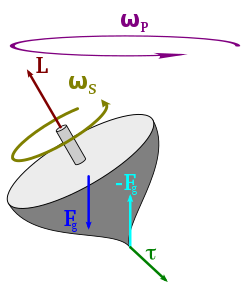Shows the angular velocity of the the spinning to around is own axis and the angular velocity of its precession. The torque is defined by action and reaction forces of gravity Fg and -Fg. The torque τ is pointing out of the paper.

The torque will cause an angular acceleration, which we can represent as a vector pointing in the same direction as the torque.

`τ = Iα`

This angular acceleration will modify the angular velocity.

`δω = αδtω' = ω + δω`

Which gives us a new angular velocity ω’, which gets tilted slightly in the direction of the torque vector. This is just the mathematical description of what I described with the space ship in orbit.

But due to the tilting always happening perpendicular to where the gravity is pulling, the weight will be shifted over causing the next torque vector to also shift. The effect of this constant shifting is what gives us the precision, where it looks like the whole spinning top is rotating slowly around an axis perpendicular to the surface, while it is rotating quickly around its own axis.

# Stability of a Gyroscope

If you look at the precession of a gyroscope you will notice it is much smaller when the gyroscope is spinning fast.

The reason for that is that with a high angular velocity, it takes a higher angular acceleration to change it noticeably.

It is the same if you consider linear movements. It is much harder to make a turn with a car at high speed than low speed.

We see the same with say orbiting spacecraft. The gravitational pull of a planet won’t alter the course of a spacecraft noticeably if it is going at high speed. If it is going at low speed it will get pulled right down on the surface of the planet.

You’ll notice this with a bike as well. You don’t fall over easily when leaning over if you are going at high speed.

So the key to gyroscope stability is a fast spinning disc. Making it heavier also helps, as it requires more force to accelerate a heavy object than a light.

# Summary

To understand a gyroscope it helps to learn about many other related phenomenon, involving rotation such as planetary orbits, bikes, spinning tops and acentrifugal governor as used of an a steam engine for instance.

In all of these cases when they seem to defy gravity it is really because there is a velocity or acceleration component which works counter to the force of gravity. Gravity will chip away at the velocity of a spinning disc, but it will take time if the disc spins very fast. And modern gyros will usually have some way of spinning them up again, using some sort of motor.

Written by